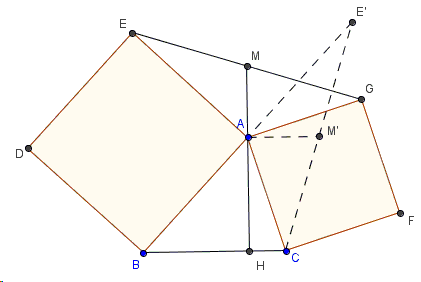# Properties of Flank Triangles - Second Proof by Symmetric Rotation

Bottema's configuration of two squares that share a vertex is naturally embedded into Vecten's configuration of three squares erected on the sides of a triangle. The latter generalizes the first of Euclid's proofs of the Pythagorean theorem, so it rightfully refers to the Bride's Chair.

In the discussion of Vecten's configuration, we proved inter alia two properties of flank triangles that are a part of Bottema's configuration.1. If $AM$ is a median in $\triangle AEG$, $AH$ is an altitude in $\triangle ABC$, then $M$, $A$, $H$ are collinear.

2. $BC = 2\cdot AM$.

The applet below illustrates one of the solutions. Use the slider at the top of the applet to rotate $\triangle AEG$ $90^{\circ}$ clockwise around $A$.

### Proof

So we rotate $\triangle AEG$ $90^{\circ}$ degrees clockwise; after the rotation, $AM'\perp AM$; $E$ maps onto $E'$, $M$ onto $M'$, $G$ onto $C$; $A$, $B$, $E'$ are collinear; $A$ the midpoint of $BE'$; $M'$ the midpoint of $CE'$. It follows that $AM'$ is the midline in $\triangle BCE'$.Therefore, $BC = 2\cdot AM'\ = 2\cdot AM$. Also $AM'\parallel BC$, implying $AM\perp BC$. In other words, $M$, $A$, $H$ are collinear.

[Honsberger, p 65] actually proves that if $M$ lies on the continuation of the altitude $AH$ then it's the midpoint of $EG$. I leave this as an exercise.

### References

1. R. Honsberger, Mathematical Diamonds, MAA, 2003### Properties of Flank Triangles